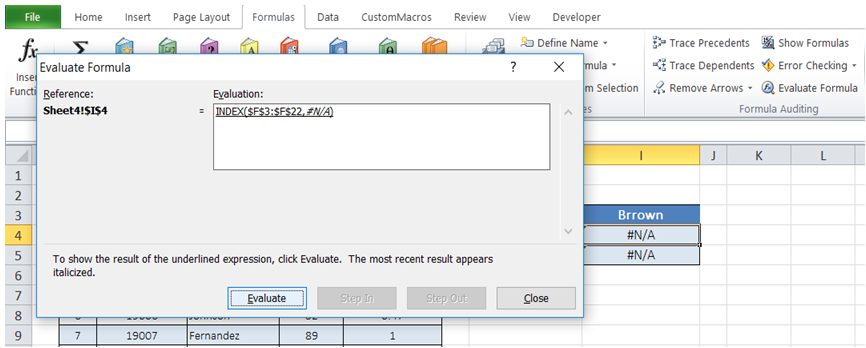# Excel Made Easy

## Trace error and step through formula in Excel

For example, in the Table below, I get "#N/A" result in 2 of the fields that I do not expect. The cells have nested formulas (Index and Match are used). In Excel, how do I step through the formula so that I can check and verify the result of each formula as part of debugging the error.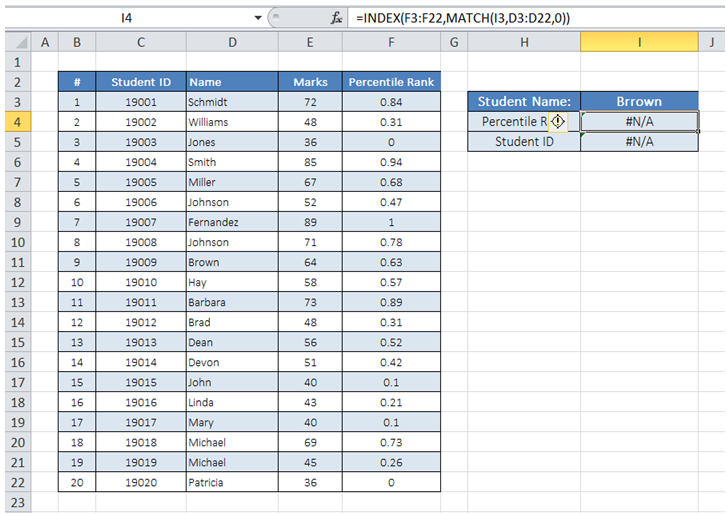To do it in Excel, here is the answer:

a) Click on "Evaluate Formula" under "Formulas".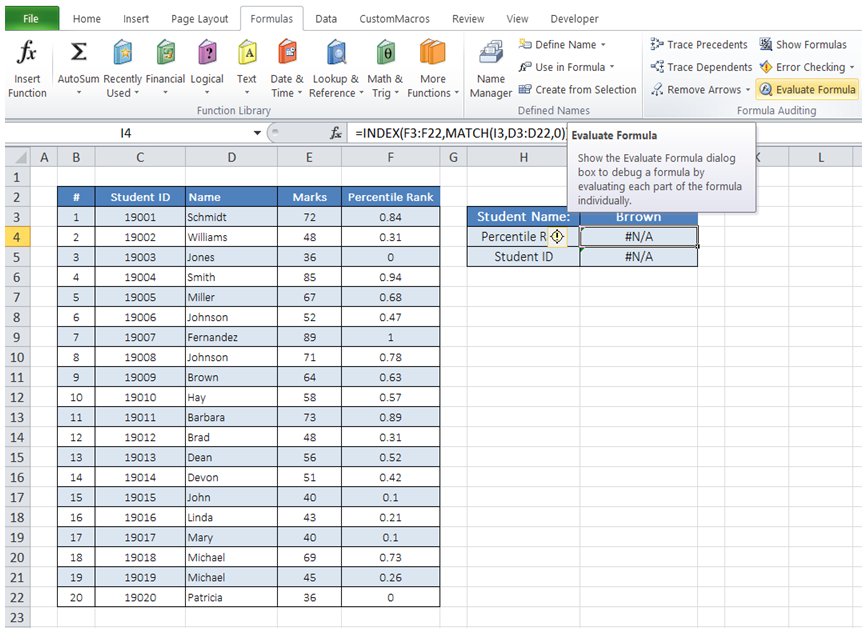b) The "Evaluate Formula" dialog box pops up. Click on "Evaluate".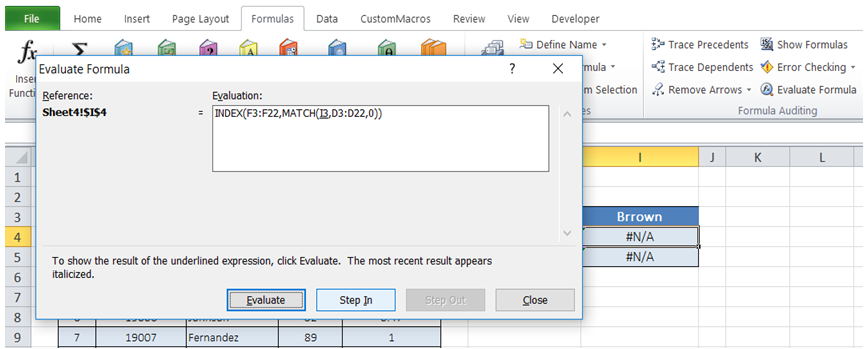c) The look up value in "MATCH" formula is updated with I3 cell content. Click on "Evaluate" again.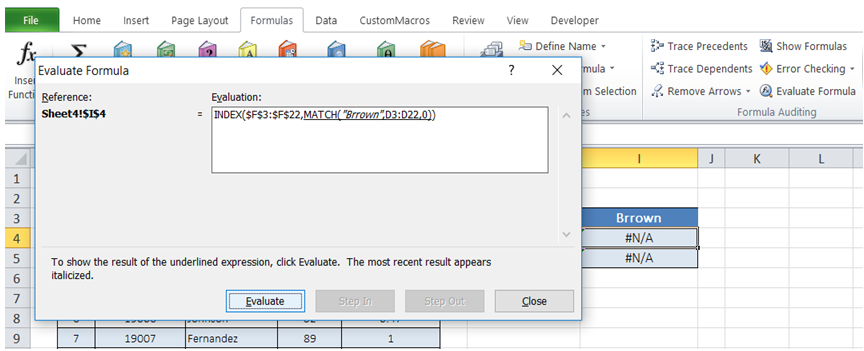d) "MATCH" formula is executed based on arguments provided and the results are returned. As can be seen from the Screenshot below, the Match formula returns #N/A indicating no match was found. Click on "Close".

Comparing the lookup value in I3 with the corresponding value in the table in D11, it is clear that the value in I3 is misspelt. Fix the incorrect value in "I3".